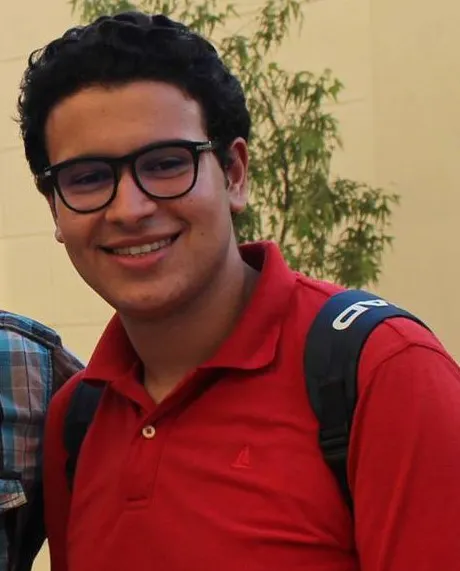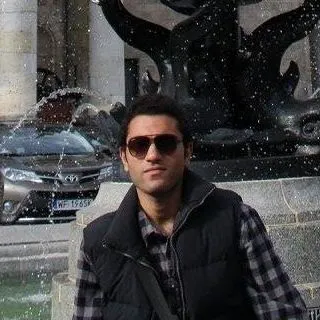# Android Controlled 6DoF Robotic Arm

Most 6DoF robotic arms come with a control board with RS-232 for desktop control, we adapt the control for use with android devices simply.

BeginnerWork in progress2 hours15,238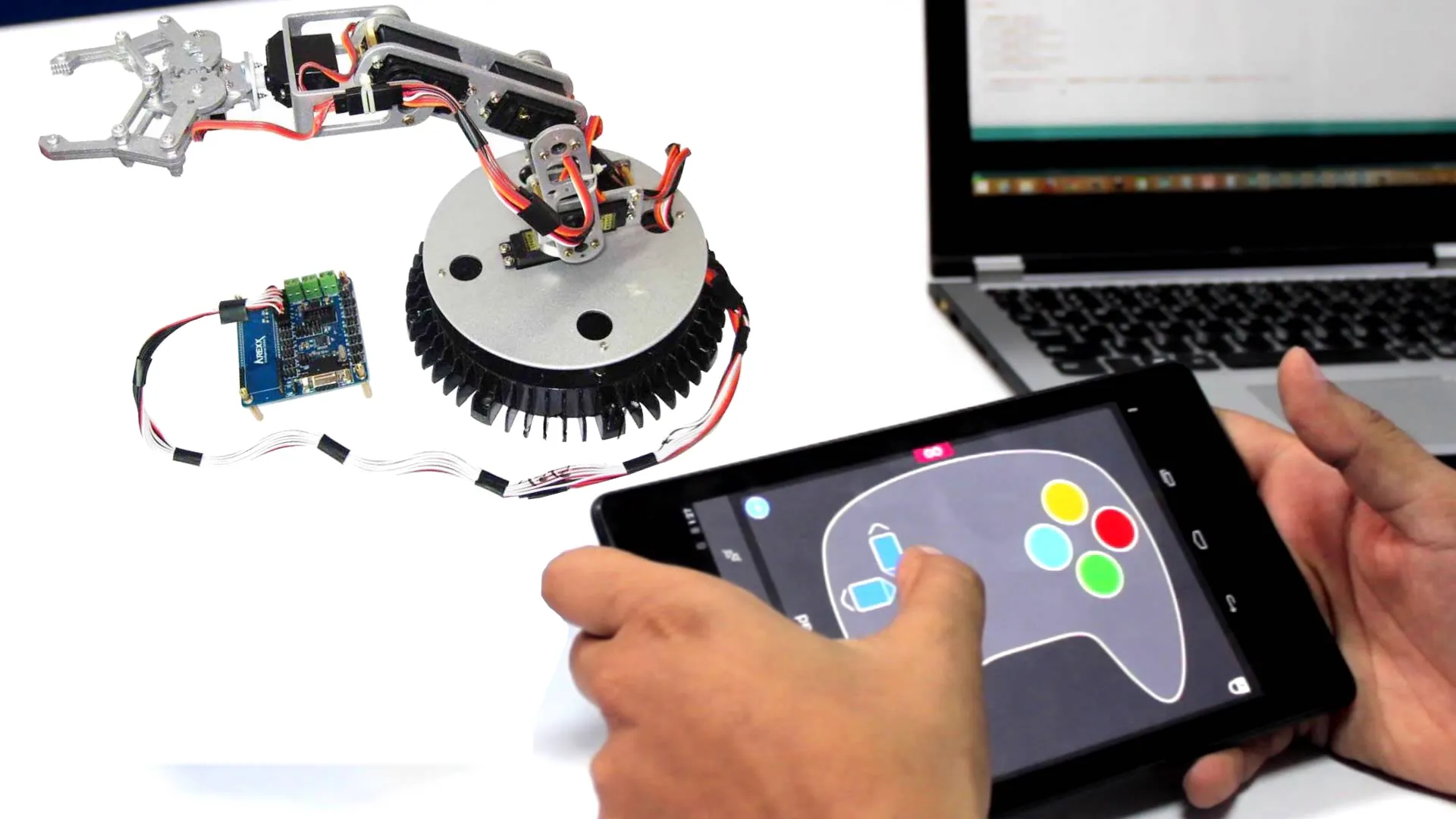## Things used in this project

### Hardware components

 DeviceHub.net 6DoF Robotic Arm
×1Arduino UNO & Genuino UNO
×1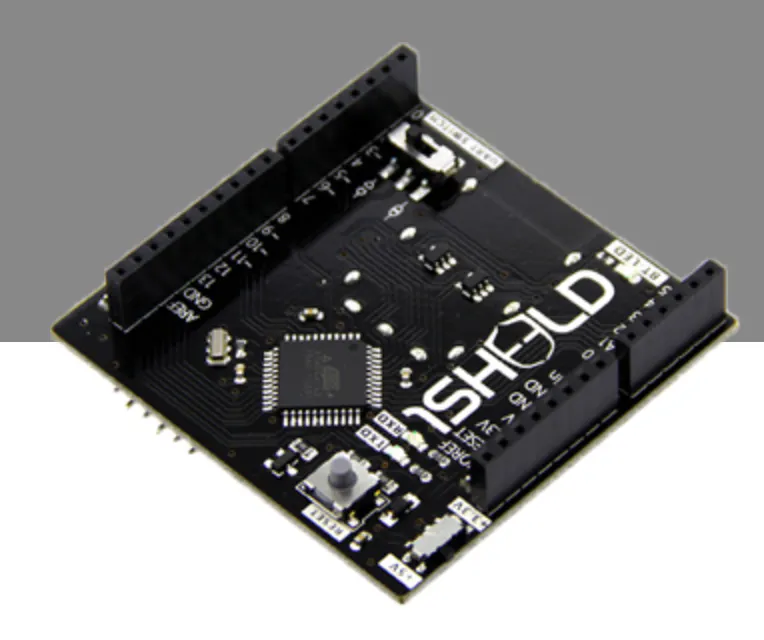1Sheeld
×1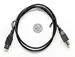USB-A to B Cable
×1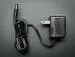9V 1A Switching Wall Power Supply
×1

### Software apps and online servicesArduino IDE

## Code

### Untitled file

Arduino
```#define CUSTOM_SETTINGS
#define INCLUDE_ACCELEROMETER_SENSOR_SHIELD

/* Include 1Sheeld library. */
#include <OneSheeld.h>
#include <Servo.h>

Servo myservo1;  // create servo object to control a servo
Servo myservo2;
Servo myservo3;
Servo myservo4;
Servo myservo5;
Servo myservo6;
int count1 = 0;
int count2 = 0;
int count3 = 0;
int count4 = 0;
int count5 = 0;

void setup()
{
/* Start communication. */
OneSheeld.begin();
myservo1.attach(3);  //the pin for the servo control
myservo2.attach(5);
myservo3.attach(6);
myservo4.attach(9);
myservo5.attach(10);
myservo6.attach(11);
}

void loop()
{

/* Turn on the LED when the red button is pressed. */
{
count3+=20;
if (count3<=180&&count3>=0){
myservo3.write(count3);
}
else if (count3>=180)
{
count3=180;
}
}
{
count3-=20;
if (count3<=180&&count3>=0){
myservo3.write(count3);
}
else if (count3<=0)
{
count3=0;
}
}

{
count4+=20;
if (count4<=180&&count4>=0){
myservo4.write(count4);
}
else if (count4>=180)
{
count4=180;
}
}
{
count4-=20;
if (count4<=180&&count4>=0){
myservo4.write(count4);
}
else if (count4<=0)
{
count4=0;
}
}

/* If up is pressed, move the car forward. */
{
count5+=20;
if (count5<=180&&count2>=0){
myservo5.write(count5);
}
else if (count5>=180)
{
count5=180;
}

}
/* If down is pressed, move the car backwards. */
{
count5-=20;
if (count5<=180&&count5>=0){
myservo1.write(count5);
}
else if (count5<=0)
{
count5=0;
}

}
/* If right is pressed, turn the car to the right. */
{
count1+=20;
if (count1<=180&&count1>=0){
myservo1.write(count1);
}
else if (count1>=180)
{
count1=180;
}
}
/* If left is pressed, turn the car to the left. */
{
count1-=20;
if (count1<=180&&count1>=0){
myservo1.write(count1);
}
else if (count1<=0)
{
count1=0;
}

}

else
{
myservo1.write(count1);    myservo3.write(count3);
myservo4.write(count4);
myservo5.write(count5);
}

if(AccelerometerSensor.getX() >=3 && AccelerometerSensor.getX()<=9)
{
myservo6.write(map(AccelerometerSensor.getX(),3,9,0,180));
}

else if (AccelerometerSensor.getY() >=-3 && AccelerometerSensor.getY()<=3)
{
myservo2.write(map(AccelerometerSensor.getY(),-3,3,0,180));
}

/* If nothing is pressed stop all motors. */
else {
myservo6.write(0);
myservo2.write(0);

}

}
```

## Credits

### ahmed barakat

1 project • 3 followers
control engineer 4th year Mansoura university interested in robotics and embedded systems worked in many projects and teams such as ROVs and Mars Rovers
Thanks to 1Sheeld.